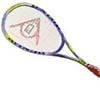#### You may also like### Rain or Shine

Predict future weather using the probability that tomorrow is wet given today is wet and the probability that tomorrow is wet given that today is dry.### Playing Squash

Playing squash involves lots of mathematics. This article explores the mathematics of a squash match and how a knowledge of probability could influence the choices you make.### Which Spinners?

Can you work out which spinners were used to generate the frequency charts?

# Squash

#### Why do this problem?

A real world problem involving conditional probability.

#### Possible approach

Half the class might work through the problem with a probability of 0.4 of winning rallies against Ivana to see whether calling 9 or 10 isadvantageous, and the other half might work on the problem with a probability of 0.3. Then a class discussion, and drawing tree diagrams of the possibilites, might help everyone to solve the problem for a general value of p and find the critical value.

#### Key question

What are the possible outcomes of the next rally, which is served by Ivana, if my probability of winning the rally is p?

What happens if Ivana loses that rally?